# Error

(diff) ← Older revision | Latest revision (diff) | Newer revision → (diff)

The difference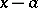, whereis a given number that is considered as the approximate value of a certain quantity with exact value. The difference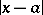is also called the absolute error. The ratio oftois called the relative error of. To characterize the error one usually states bounds on it. A numbersuch that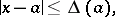is called a bound on the absolute error. A number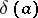such that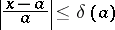is called a bound on the relative error. A bound on the relative error is frequently expressed in percentages. The smallest possible numbers are taken as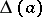and.

The information that the numberis the approximate value ofwith a boundof the absolute error is usually stated as: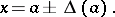The analogous relation for the relative error is written as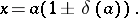The bounds on the absolute and relative errors indicate the maximum possible discrepancy betweenand. At the same time, one often uses error characteristics that incorporate the character of the error (for example, the error of a measurement) and the frequencies of different values of the difference betweenand. Probabilistic methods are used in the last approach (see Errors, theory of).

In the numerical solution of a problem, the error in the result is due to inaccuracies occurring in the formulation and in the methods of solution. The error arising because of inaccuracy in the mathematical description of a real process is called the error of the model; that arising from inaccuracy in specifying the initial data is called the input-data error; that due to inaccuracy in the method of solution is called the methodological error; and that due to inaccuracy in the computations is called the computational error (rounding error). Sometimes the error of the model and the input-data error are combined under the name inherent error.

During the calculations, the initial errors are sequentially transferred from operation to operation, and they accumulate and generate new errors. Error occurrence and propagation in calculations is the subject of special researches (see Computational mathematics).

How to Cite This Entry:
Error. Encyclopedia of Mathematics. URL: http://encyclopediaofmath.org/index.php?title=Error&oldid=17295
This article was adapted from an original article by G.D. Kim (originator), which appeared in Encyclopedia of Mathematics - ISBN 1402006098. See original article